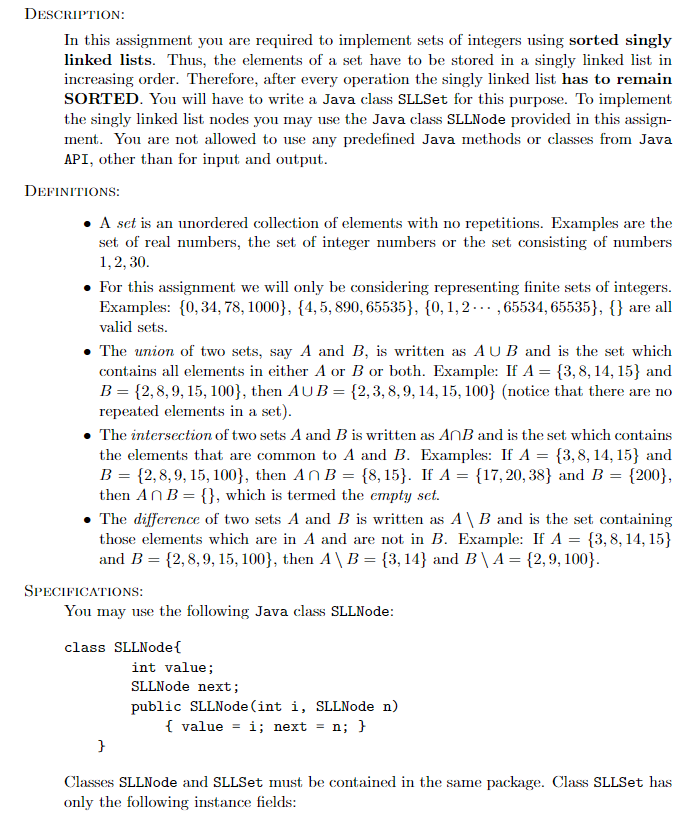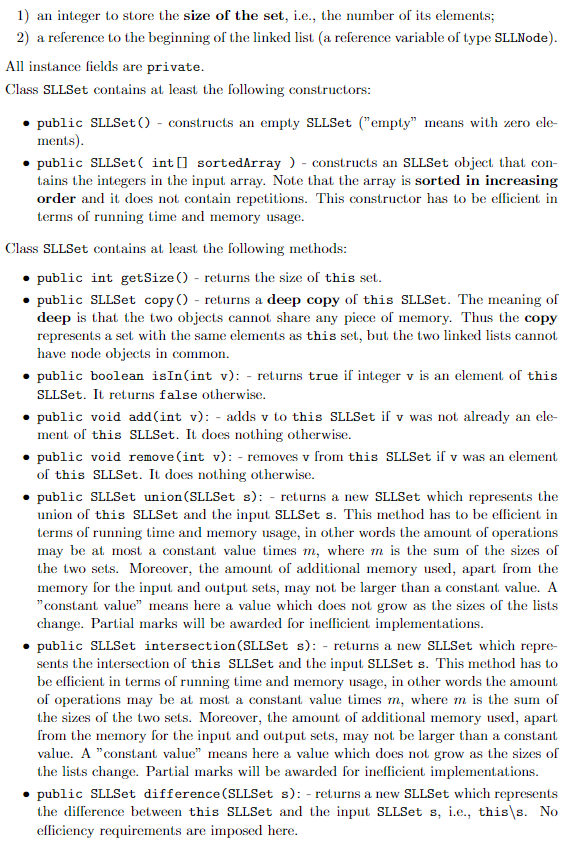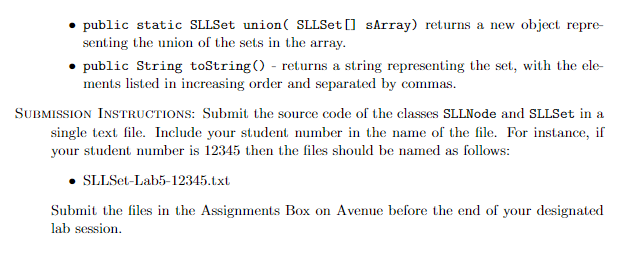# (Solved) : Screenshots Question Coming Text Code Explain Algorithms Statements Used Comments Thanks P Q42711958 . . .These screenshots are the question and the comingtext is the code for it all you have to do is explain all thealgorithms and statements used as comments,Thanks!!

public class SLLSet {
private int size;

public SLLSet(){
size = 0;
}

public SLLSet(int[] sortedArray){
if(sortedArray.length > 0){
int i;
SLLNode p = new SLLNode(sortedArray,null);
size = sortedArray.length;
for(i = 1;i<sortedArray.length;i++){
p.next = new SLLNode(sortedArray[i],null);
p = p.next;
}
}
else{
size = 0;
}
}//End of SLLSet constructor

public int getSize(){
return size;
}//Returns the size of the list

public SLLSet copy(){
int[] list = new int[size];
int i = 0;
while(p != null){
list[i] = p.value;
p = p.next;
i++;
}
SLLSet s = new SLLSet(list);
s.size = size;
return s;
}//End of copy function

public boolean isIn(int v){
while(p != null){
if(p.next == null){
break;
}
if(p.value == v){
return true;
}
p = p.next;
}
return false;
}//End of isIn

if(this.isIn(v) == false){
}
}
else{
int x;
while(p.next != null){
x = p.next.value;
if(v < x && v > p.value){
p.next = new SLLNode(v,p.next);
}
p = p.next;
}
if(v > p.value){
p.next = new SLLNode(v,null);
}
}
size++;
}

public void remove(int v){
if(isIn(v)){
size–;
if(p.value == v){
}
else{
while(p != null){
if(p.next == null){
break;
}
else if(p.next.value == v){
p.next = p.next.next;
break;
}
p = p.next;
}
}
}
}

public SLLSet union(SLLSet s){
SLLSet union = this.copy();
while(j != null){
j = j.next;
}
}
return union;
}

public SLLSet intersection(SLLSet s){
SLLSet inter = new SLLSet();
if(q != null || p != null){
while(p != null){
while(q != null){
if(p.value == q.value){
}
q = q.next;
}
p = p.next;
}
}
return inter;
}

public SLLSet difference(SLLSet s){
SLLSet diff = this.union(s);
while(p != null){
if(s.isIn(p.value)){
diff.remove(p.value);
}
p = p.next;
}
while(p != null){
if(this.isIn(p.value)){
diff.remove(p.value);
}
p = p.next;
}
return diff;
}

public static SLLSet union(SLLSet[] sArray){
int i;
SLLSet uni = new SLLSet();
//SLLSet uni = sArray.copy();
for(i = 0;i<sArray.length;i++){
uni = uni.union(sArray[i]);
}
return uni;
}

public String toString(){
String s;
s = “(“;
while(p != null){
s += p.value;
p = p.next;
if(p != null){
s += “,”;
}
}
s += “)”;
return s;
}//Prints out the nodes
}

1) an integer to store the size of the set, i.e., the number of its elements; 2) a reference to the beginning of the linked list (a reference variable of type SLLNode). All instance fields are private. Class SLLSet contains at least the following constructors: • public SLLSet() – constructs an empty SLLSet (“empty” means with zero ele ments). • public SLLSet(int[] sortedArray ) – constructs an SLLSet object that con- tains the integers in the input array. Note that the array is sorted in increasing order and it does not contain repetitions. This constructor has to be efficient in terms of running time and memory usage. Class SLLSet contains at least the following methods: • public int getSize() – returns the size of this set. • public SLLSet copy() – returns a deep copy of this SLLSet. The meaning of deep is that the two objects cannot share any piece of memory. Thus the copy represents a set with the same elements as this set, but the two linked lists cannot have node objects in common. • public boolean isIn(int v): – returns true if integer v is an element of this SLLSet. It returns false otherwise. • public void add (int v): – adds v to this SLLSet if v was not already an ele ment of this SLLSet. It does nothing otherwise. • public void remove(int v): – removes v from this SLLSet il v was an element of this SLLSet. It does nothing otherwise. • public SLLSet union (SLLSet s): – returns a new SLLSet which represents the union of this SLLSet and the input SLLSet s. This method has to be ellicient in terms of running time and memory usage, in other words the amount of operations may be at most a constant value times m, where m is the sum of the sizes of the two sets. Moreover, the amount of additional memory used, apart from the memory for the input and output sets, may not be larger than a constant value. A “constant value” means here a value which does not grow as the sizes of the lists change. Partial marks will be awarded for inefficient implementations. • public SLLSet intersection (SLLSet s): – returns a new SLLSet which repre- sents the intersection of this SLLSet and the input SLLSet s. This method has to be efficient in terms of running time and memory usage, in other words the amount of operations may be at most a constant value times m, where m is the sum of the sizes of the two sets. Moreover, the amount of additional memory used, apart from the memory for the input and output sets, may not be larger than a constant value. A constant value” means here a value which does not grow as the sizes of the lists change. Partial marks will be awarded for inefficient implementations. • public SLLSet difference (SLLSet s): – returns a new SLLSet which represents the difference between this SLLSet and the input SLLSet s, i.e., thiss. No elliciency requirements are imposed here.
• public static SLLSet union( SLLSet [] sArray) returns a new object repre senting the union of the sets in the array. • public String toString() – returns a string representing the set, with the ele ments listed in increasing order and separated by commas. SUBMISSION INSTRUCTIONS: Submit the source code of the classes SLLNode and SLLSet in a single text file. Include your student number in the name of the file. For instance, if your student number is 12345 then the files should be named as follows: • SLLSet-Lab5-12345.txt Submit the files in the Assignments Box on Avenue before the end of your designated lab session.

Answer to These screenshots are the question and the coming text is the code for it all you have to do is explain all the algorith…

We are the best freelance writing portal. Looking for online writing, editing or proofreading jobs? We have plenty of writing assignments to handle.